# interpolateSolution

Interpolate PDE solution to arbitrary points

## Syntax

``uintrp = interpolateSolution(results,xq,yq)``
``uintrp = interpolateSolution(results,xq,yq,zq)``
``uintrp = interpolateSolution(results,querypoints)``
``uintrp = interpolateSolution(___,iU)``
``uintrp = interpolateSolution(___,iT)``

## Description

example

````uintrp = interpolateSolution(results,xq,yq)` returns the interpolated values of the solution to the scalar stationary equation specified in `results` at the 2-D points specified in `xq` and `yq`.```

example

````uintrp = interpolateSolution(results,xq,yq,zq)` returns the interpolated values at the 3-D points specified in `xq`, `yq`, and `zq`.```

example

````uintrp = interpolateSolution(results,querypoints)` returns the interpolated values at the points in `querypoints`.```

example

````uintrp = interpolateSolution(___,iU)`, for any previous syntax, returns the interpolated values of the solution to the system of stationary equations for equation indices `iU`.```

example

````uintrp = interpolateSolution(___,iT)` returns the interpolated values of the solution to the time-dependent or eigenvalue equation or system of such equations at times or modal indices `iT`. For a system of time-dependent or eigenvalue equations, specify both time/modal indices `iT` and equation indices `iU````

## Examples

collapse all

Interpolate the solution to a scalar problem along a line and plot the result.

Create the solution to the problem $-\Delta u=1$ on the L-shaped membrane with zero Dirichlet boundary conditions.

```model = createpde; geometryFromEdges(model,@lshapeg); applyBoundaryCondition(model,"dirichlet", ... "Edge",1:model.Geometry.NumEdges, ... "u",0); specifyCoefficients(model,"m",0,... "d",0,... "c",1,... "a",0,... "f",1); generateMesh(model,"Hmax",0.05); results = solvepde(model);```

Interpolate the solution along the straight line from `(x,y) = (-1,-1)` to `(1,1)`. Plot the interpolated solution.

```xq = linspace(-1,1,101); yq = xq; uintrp = interpolateSolution(results,xq,yq); plot(xq,uintrp) xlabel("x") ylabel("u(x)")```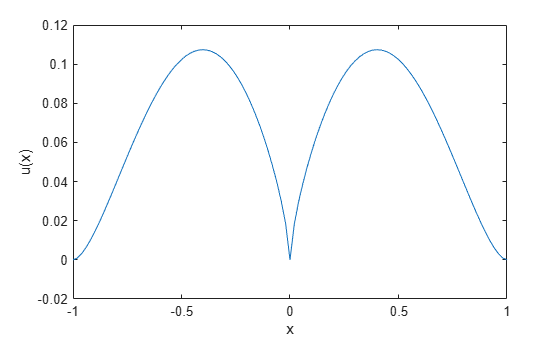Calculate the mean exit time of a Brownian particle from a region that contains absorbing (escape) boundaries and reflecting boundaries. Use the Poisson's equation with constant coefficients and 3-D rectangular block geometry to model this problem.

Create the solution for this problem.

```model = createpde; importGeometry(model,"Block.stl"); applyBoundaryCondition(model,"dirichlet","Face",[1,2,5],"u",0); specifyCoefficients(model,"m",0,... "d",0,... "c",1,... "a",0,... "f",2); generateMesh(model); results = solvepde(model);```

Create a grid and interpolate the solution to the grid.

```[X,Y,Z] = meshgrid(0:135,0:35,0:61); uintrp = interpolateSolution(results,X,Y,Z); uintrp = reshape(uintrp,size(X));```

Create a contour slice plot for five fixed values of the `y` coordinate.

```contourslice(X,Y,Z,uintrp,[],0:4:16,[]) colormap jet xlabel("x") ylabel("y") zlabel("z") xlim([0,100]) ylim([0,20]) zlim([0,50]) axis equal view(-50,22) colorbar```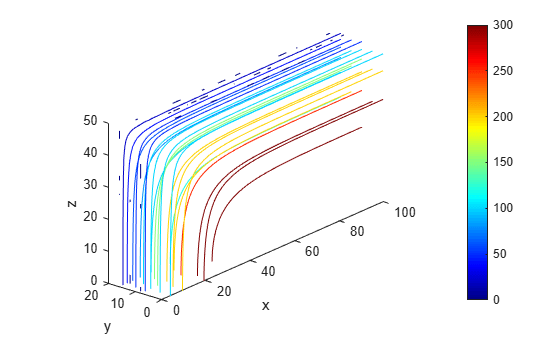Solve a scalar stationary problem and interpolate the solution to a dense grid.

Create the solution to the problem $-\Delta u=1$ on the L-shaped membrane with zero Dirichlet boundary conditions.

```model = createpde; geometryFromEdges(model,@lshapeg); applyBoundaryCondition(model,"dirichlet", ... "Edge",1:model.Geometry.NumEdges, ... "u",0); specifyCoefficients(model,"m",0,"d",0,"c",1,"a",0,"f",1); generateMesh(model,"Hmax",0.05); results = solvepde(model);```

Interpolate the solution on the grid from –1 to 1 in each direction.

```v = linspace(-1,1,101); [X,Y] = meshgrid(v); querypoints = [X(:),Y(:)]'; uintrp = interpolateSolution(results,querypoints);```

Plot the resulting interpolation on a mesh.

```uintrp = reshape(uintrp,size(X)); mesh(X,Y,uintrp) xlabel("x") ylabel("y")```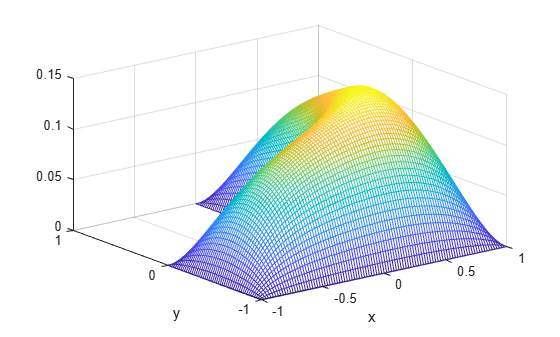Create the solution to a two-component system and plot the two components along a planar slice through the geometry.

Create a PDE model for two components. Import the geometry of a torus.

```model = createpde(2); importGeometry(model,"Torus.stl"); pdegplot(model,"FaceLabels","on");```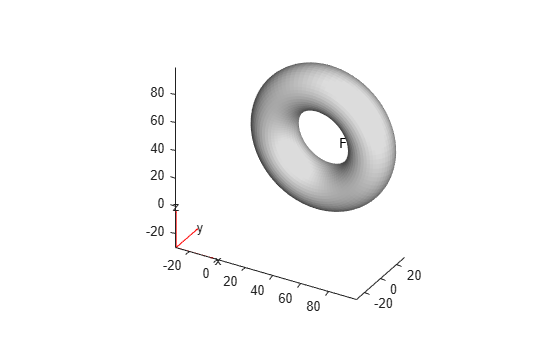Set boundary conditions.

```gfun = @(region,state)[0,region.z-40]; applyBoundaryCondition(model,"neumann","Face",1,"g",gfun); ufun = @(region,state)[region.x-40,0]; applyBoundaryCondition(model,"dirichlet","Face",1,"u",ufun);```

Set the problem coefficients.

```specifyCoefficients(model,"m",0,... "d",0,... "c",[1;0;1;0;0;1;0;0;1;0;1; 0;1;0;0;1;0;1;0;0;1],... "a",0,... "f",[1;1]);```

Create a mesh and solve the problem.

```generateMesh(model); results = solvepde(model);```

Interpolate the results on a plane that slices the torus for each of the two components.

```[X,Z] = meshgrid(0:100); Y = 15*ones(size(X)); uintrp = interpolateSolution(results,X,Y,Z,[1,2]);```

Plot the two components.

```sol1 = reshape(uintrp(:,1),size(X)); sol2 = reshape(uintrp(:,2),size(X)); figure surf(X,Z,sol1) title("Component 1")```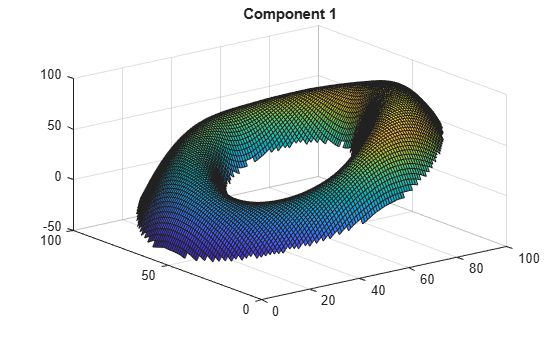```figure surf(X,Z,sol2) title("Component 2")```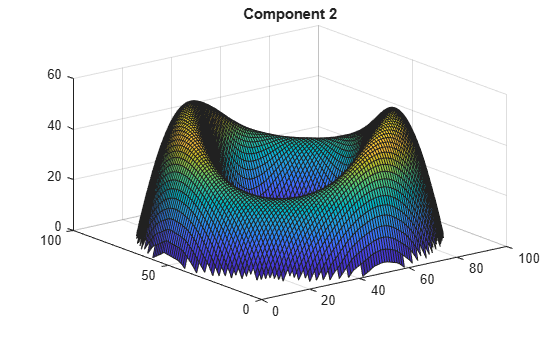Solve a scalar eigenvalue problem and interpolate one eigenvector to a grid.

Find the eigenvalues and eigenvectors for the L-shaped membrane.

```model = createpde(1); geometryFromEdges(model,@lshapeg); applyBoundaryCondition(model,"dirichlet", ... "Edge",1:model.Geometry.NumEdges, ... "u",0); specifyCoefficients(model,"m",0,... "d",1,... "c",1,... "a",0,... "f",0); r = [0,100]; generateMesh(model,"Hmax",1/50); results = solvepdeeig(model,r);```
``` Basis= 10, Time= 0.39, New conv eig= 0 Basis= 11, Time= 0.42, New conv eig= 0 Basis= 12, Time= 0.44, New conv eig= 0 Basis= 13, Time= 0.46, New conv eig= 0 Basis= 14, Time= 0.48, New conv eig= 0 Basis= 15, Time= 0.50, New conv eig= 0 Basis= 16, Time= 0.51, New conv eig= 1 Basis= 17, Time= 0.53, New conv eig= 1 Basis= 18, Time= 0.55, New conv eig= 1 Basis= 19, Time= 0.56, New conv eig= 1 Basis= 20, Time= 0.59, New conv eig= 1 Basis= 21, Time= 0.61, New conv eig= 2 Basis= 22, Time= 0.63, New conv eig= 3 Basis= 23, Time= 0.65, New conv eig= 3 Basis= 24, Time= 0.66, New conv eig= 3 Basis= 25, Time= 0.69, New conv eig= 3 Basis= 26, Time= 0.69, New conv eig= 3 Basis= 27, Time= 0.71, New conv eig= 3 Basis= 28, Time= 0.81, New conv eig= 3 Basis= 29, Time= 0.89, New conv eig= 5 Basis= 30, Time= 0.95, New conv eig= 5 Basis= 31, Time= 0.98, New conv eig= 5 Basis= 32, Time= 1.01, New conv eig= 5 Basis= 33, Time= 1.07, New conv eig= 5 Basis= 34, Time= 1.14, New conv eig= 5 Basis= 35, Time= 1.16, New conv eig= 5 Basis= 36, Time= 1.18, New conv eig= 5 Basis= 37, Time= 1.19, New conv eig= 6 Basis= 38, Time= 1.22, New conv eig= 6 Basis= 39, Time= 1.24, New conv eig= 7 Basis= 40, Time= 1.26, New conv eig= 7 Basis= 41, Time= 1.30, New conv eig= 7 Basis= 42, Time= 1.33, New conv eig= 8 Basis= 43, Time= 1.34, New conv eig= 7 Basis= 44, Time= 1.37, New conv eig= 7 Basis= 45, Time= 1.39, New conv eig= 8 Basis= 46, Time= 1.42, New conv eig= 9 Basis= 47, Time= 1.46, New conv eig= 9 Basis= 48, Time= 1.51, New conv eig= 10 Basis= 49, Time= 1.55, New conv eig= 11 Basis= 50, Time= 1.58, New conv eig= 13 Basis= 51, Time= 1.60, New conv eig= 14 Basis= 52, Time= 1.63, New conv eig= 14 Basis= 53, Time= 1.67, New conv eig= 14 Basis= 54, Time= 1.70, New conv eig= 14 Basis= 55, Time= 1.72, New conv eig= 14 Basis= 56, Time= 1.75, New conv eig= 14 Basis= 57, Time= 1.78, New conv eig= 14 Basis= 58, Time= 1.80, New conv eig= 14 Basis= 59, Time= 1.85, New conv eig= 15 Basis= 60, Time= 1.88, New conv eig= 15 Basis= 61, Time= 1.93, New conv eig= 15 Basis= 62, Time= 1.95, New conv eig= 16 Basis= 63, Time= 1.98, New conv eig= 16 Basis= 64, Time= 2.06, New conv eig= 16 Basis= 65, Time= 2.10, New conv eig= 17 Basis= 66, Time= 2.12, New conv eig= 18 Basis= 67, Time= 2.14, New conv eig= 18 Basis= 68, Time= 2.17, New conv eig= 19 Basis= 69, Time= 2.20, New conv eig= 19 Basis= 70, Time= 2.24, New conv eig= 19 Basis= 71, Time= 2.27, New conv eig= 20 Basis= 72, Time= 2.29, New conv eig= 20 Basis= 73, Time= 2.31, New conv eig= 20 Basis= 74, Time= 2.34, New conv eig= 20 Basis= 75, Time= 2.35, New conv eig= 21 Basis= 76, Time= 2.40, New conv eig= 22 End of sweep: Basis= 76, Time= 2.40, New conv eig= 22 Basis= 32, Time= 2.62, New conv eig= 0 Basis= 33, Time= 2.65, New conv eig= 0 Basis= 34, Time= 2.68, New conv eig= 0 Basis= 35, Time= 2.69, New conv eig= 0 Basis= 36, Time= 2.70, New conv eig= 0 Basis= 37, Time= 2.72, New conv eig= 0 Basis= 38, Time= 2.74, New conv eig= 0 Basis= 39, Time= 2.75, New conv eig= 0 Basis= 40, Time= 2.77, New conv eig= 0 Basis= 41, Time= 2.81, New conv eig= 0 Basis= 42, Time= 2.83, New conv eig= 0 Basis= 43, Time= 2.84, New conv eig= 0 Basis= 44, Time= 2.86, New conv eig= 0 Basis= 45, Time= 2.88, New conv eig= 0 Basis= 46, Time= 2.90, New conv eig= 0 End of sweep: Basis= 46, Time= 2.90, New conv eig= 0 ```

Interpolate the eigenvector corresponding to the fifth eigenvalue to a coarse grid and plot the result.

```[xq,yq] = meshgrid(-1:0.1:1); uintrp = interpolateSolution(results,xq,yq,5); uintrp = reshape(uintrp,size(xq)); surf(xq,yq,uintrp)```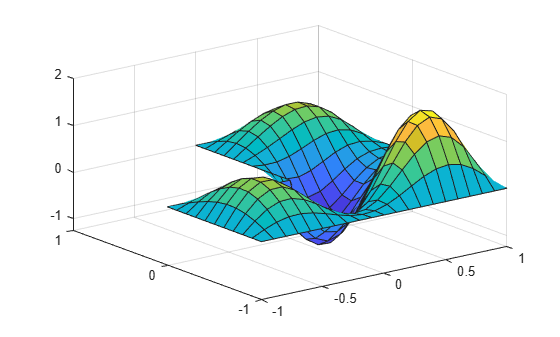Solve a system of time-dependent PDEs and interpolate the solution.

Import slab geometry for a 3-D problem with three solution components. Plot the geometry.

```model = createpde(3); importGeometry(model,"Plate10x10x1.stl"); pdegplot(model,"FaceLabels","on","FaceAlpha",0.5)```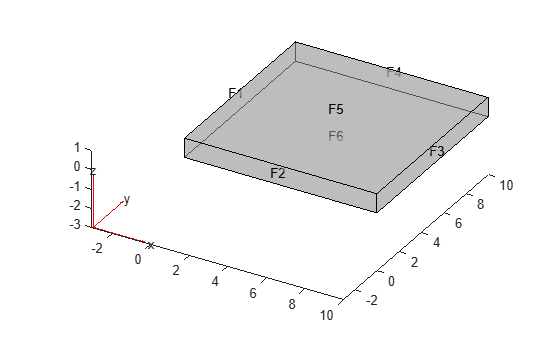Set boundary conditions such that face 2 is fixed (zero deflection in any direction) and face 5 has a load of `1e3` in the positive `z`-direction. This load causes the slab to bend upward. Set the initial condition that the solution is zero, and its derivative with respect to time is also zero.

```applyBoundaryCondition(model,"dirichlet","Face",2,"u",[0,0,0]); applyBoundaryCondition(model,"neumann","Face",5,"g",[0,0,1e3]); setInitialConditions(model,0,0);```

Create PDE coefficients for the equations of linear elasticity. Set the material properties to be similar to those of steel. See Linear Elasticity Equations.

```E = 200e9; nu = 0.3; specifyCoefficients(model,"m",1,... "d",0,... "c",elasticityC3D(E,nu),... "a",0,... "f",[0;0;0]);```

Generate a mesh, setting `Hmax` to 1.

`generateMesh(model,"Hmax",1);`

Solve the problem for times 0 through `5e-3` in steps of `1e-4`.

```tlist = 0:1e-4:5e-3; results = solvepde(model,tlist);```

Interpolate the solution at fixed `x`- and `z`-coordinates in the centers of their ranges, 5 and 0.5 respectively. Interpolate for `y` from 0 through 10 in steps of 0.2. Obtain just component 3, the `z`-component of the solution.

```yy = 0:0.2:10; zz = 0.5*ones(size(yy)); xx = 10*zz; component = 3; uintrp = interpolateSolution(results,xx,yy,zz, ... component,1:length(tlist));```

The solution is a 51-by-1-by-51 array. Use `squeeze` to remove the singleton dimension. Removing the singleton dimension transforms this array to a 51-by-51 matrix which simplifies indexing into it.

`uintrp = squeeze(uintrp);`

Plot the solution as a function of `y` and time.

```[X,Y] = ndgrid(yy,tlist); figure surf(X,Y,uintrp) xlabel("Y") ylabel("Time") title("Deflection at x = 5, z = 0.5") zlim([0,14e-5])```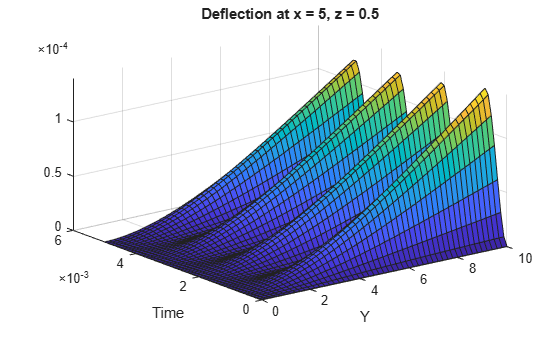## Input Arguments

collapse all

PDE solution, specified as a `StationaryResults` object, a `TimeDependentResults` object, or an `EigenResults` object. Create `results` using `solvepde`, `solvepdeeig`, or `createPDEResults`.

Example: `results = solvepde(model)`

x-coordinate query points, specified as a real array. `interpolateSolution` evaluates the solution at the 2-D coordinate points `[xq(i),yq(i)]` or at the 3-D coordinate points `[xq(i),yq(i),zq(i)]`. So `xq`, `yq`, and (if present) `zq` must have the same number of entries.

`interpolateSolution` converts query points to column vectors `xq(:)`, `yq(:)`, and (if present) `zq(:)`. The returned solution is a column vector of the same size. To ensure that the dimensions of the returned solution is consistent with the dimensions of the original query points, use `reshape`. For example, use ```uintrp = reshape(gradxuintrp,size(xq))```.

Data Types: `double`

y-coordinate query points, specified as a real array. `interpolateSolution` evaluates the solution at the 2-D coordinate points `[xq(i),yq(i)]` or at the 3-D coordinate points `[xq(i),yq(i),zq(i)]`. So `xq`, `yq`, and (if present) `zq` must have the same number of entries. Internally, `interpolateSolution` converts query points to the column vector `yq(:)`.

Data Types: `double`

z-coordinate query points, specified as a real array. `interpolateSolution` evaluates the solution at the 3-D coordinate points `[xq(i),yq(i),zq(i)]`. So `xq`, `yq`, and `zq` must have the same number of entries. Internally, `interpolateSolution` converts query points to the column vector `zq(:)`.

Data Types: `double`

Query points, specified as a real matrix with either two rows for 2-D geometry, or three rows for 3-D geometry. `interpolateSolution` evaluates the solution at the coordinate points `querypoints(:,i)`, so each column of `querypoints` contains exactly one 2-D or 3-D query point.

Example: For 2-D geometry, ```querypoints = [0.5,0.5,0.75,0.75; 1,2,0,0.5]```

Data Types: `double`

Equation indices, specified as a vector of positive integers. Each entry in `iU` specifies an equation index.

Example: `iU = [1,5]` specifies the indices for the first and fifth equations.

Data Types: `double`

Time or mode indices, specified as a vector of positive integers. Each entry in `iT` specifies a time index for time-dependent solutions, or a mode index for eigenvalue solutions.

Example: `iT = 1:5:21` specifies the time or mode for every fifth solution up to 21.

Data Types: `double`

## Output Arguments

collapse all

Solution at query points, returned as an array. For query points that are outside the geometry, `uintrp` = `NaN`. For details about dimensions of the solution, see Dimensions of Solutions, Gradients, and Fluxes.

## Version History

Introduced in R2015b